Question

Sally finds herself stranded on a frozen pond so slippery that she can’t stand up or walk on it. To save herself, she throws one of her heavy boots horizontally, directly away from the closest shore. Sally’s mass is 60.0 kg, the boot’s mass is 5.0 kg, and Sally throws the boot with speed equal to 30.0 m/s. For all parts, assume the ice is frictionless. 1) What is Sally’s speed immediately after throwing the bo

1.a) 2.5 m/s. (In the opposite direction to the direction in which she threw the boot).

b) The centre of mass is still at the starting point for both bodies.

c) It’ll take Sally 12 s to reach the shore which is 30 m from her starting point.

Explanation:

Linear momentum is conserved.

(mass of boot) × (velocity of boot) + (mass of sally) × (velocity of Sally) = 0

5×30 + 60 × v = 0

v = (-150/60) = -2.5 m/s. (Minus inicates that motion is in the opposite direction to the direction in which she threw the boot).

b) At time t = 10 s,

Sally has travelled 25 m and the boot has travelled 300 m.

Taking the starting point for both bodies as the origin, and Sally’s direction as the positive direction.

Centre of mass = [(60)(25) + (5)(-300)]/(60+5)

= 0 m.

The centre of mass is still at the starting point for both bodies.

c) The shore is 30 m away.

Speed = (Distance)/(time)

Time = (Distance)/(speed) = (30/2.5)

Time = 12 s

Hope this Helps!!!

2.Given Information:

mass of boot = m₁ = 5.0 kg

mass of Sally = m₂ = 60.0 kg

speed of boot = v₁ = 30.0 m/s

Required Information:

speed of Sally = v₂ = ?

speed of Sally =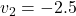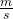Explanation:

Conservation of Momentum:

The total momentum of the system is conserved when there is no net external force is acting on the system.

Mathematically,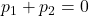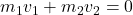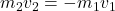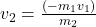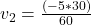The negative sign indicates that the velocity of Sally is in the opposite direction as compared to the velocity of boot.

Therefore, Sally’s speed immediately after throwing the boot is -2.5 m/s